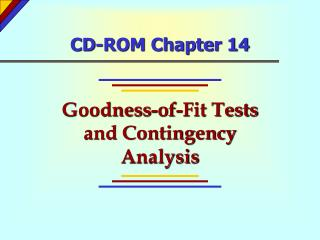DownloadDownload PresentationCD-ROM Chapter 14

# CD-ROM Chapter 14

Download Presentation## CD-ROM Chapter 14

- - - - - - - - - - - - - - - - - - - - - - - - - - - E N D - - - - - - - - - - - - - - - - - - - - - - - - - - -
##### Presentation Transcript

1. CD-ROM Chapter 14 Goodness-of-Fit Tests and Contingency Analysis

2. Chapter 14 - Chapter Outcomes After studying the material in this chapter, you should be able to: • Utilize the chi-square goodness-of-fit test to determine whether data from a process fit a specified distribution. • Set up a contingency analysis table and perform a chi-square test of independence.

3. Chi-Square Goodness-of-Fit Test CHI-SQUARE GOODNESS OF FIT TEST STATISTIC where: k = Number of categories oi = Observed cell frequency for category i ei = Expected cell frequency for category i

4. Chi-Square Goodness-of-Fit Test(Vista Health Guard Example) H0: Patient demand is evenly spread throughout the weekdays and 25% lower on weekends. HA: Patient demand follows some other distribution.  = 0.05

5. Chi-Square Goodness-of-Fit Test(Figure 14-3)

6. Chi-Square Goodness-of-Fit Test(Figure 14-3) f(2) d.f. = k - 1 = 7 - 1 = 6 Rejection Region  = 0.05 2 2 = 12.592 Decision Rule: If 2 > 12.592, reject H0 Otherwise, do not reject H0

7. Chi-Square Goodness-of-Fit Test(Figure 14-3) Since 3,302.7 > 12.592, reject H0

8. Contingency Analysis A contingency table is a table used to classify sample observations according to two or more identifiable characteristics. Also called a crosstabulation table.

9. Contingency Analysis CHI-SQUARE CONTINGENCY TEST STATISTIC where: oij = Observed frequency in cell (i, j) eij = Expected frequency in cell (i, j) r = Number of rows c = Number of columns

10. Contingency Analysis(From Figure 14-9) H0: Gender of yearbook editor is independent of college’s funding source. HA: Gender of yearbook editor is not independent of college’s funding source.  = 0.05 Private Public Actual = 14 Actual = 43 Male Expected = 39.98 Expected = 17.02 Actual = 141 Actual = 23 Female Expected = 115.02 Expected = 48.98

11. Contingency Analysis(From Figure 14-9) f(2) d.f. = (r - 1)(c - 1) = (1)(1) = 1 Rejection Region  = 0.05 2 2 = 3.841 Decision Rule: If 2 > 3.841, reject H0 Otherwise, do not reject H0

12. Contingency Analysis(From Figure 14-9) Test Statistic: Since 76.188 > 3.841, reject H0

13. Contingency Analysis EXPECTED CELL FREQUENCIES

14. • Chi-Square Goodness-of-Fit test • Contingency Table Key Terms